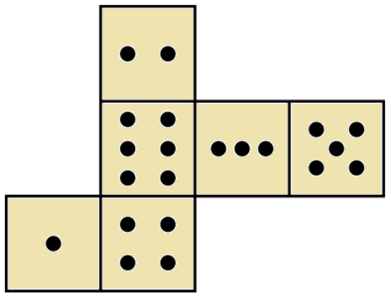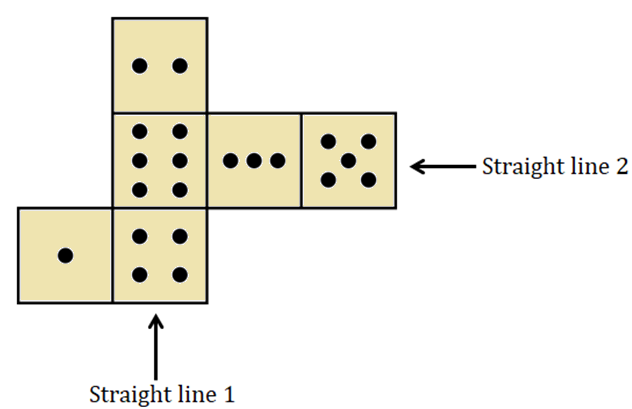Question of The Day11-11-2019

If the following figure is folded from the intersection of common edges to make dice, then how many dots will be on the face opposite to the face having 1 dot?Correct Answer : d ) 3

Explanation :

These types of questions can be solved using the logic given below:

• In the 2D question figure if there are two faces or squares in a straight line, then the two faces will never be opposite to each other in the final 3D cube.
• In the 2D question figure, if there are more than 2 faces or squares in a straight line, then the faces or squares at a gap of one block in the straight line will always be opposite to each other.

Now, considering the given figure:In straight-line 1, face having 4 dots and face having 2 dots are at a gap of one face so, they will be opposite to each other.

In straight-line 2, face having 6 dots and face having 5 dots are at a gap of one face so, they will be opposite to each other.

Therefore, the remaining faces, i.e. face having 1 dot and face having 3 dots, will be opposite to each other.

Hence, (d) is the correct answer.

Such type of questions is asked in various exams like SSC CGL, SSC MTS, SSC CPO, SSC CHSL, RRB JE, RRB NTPC, RRB GROUP D, etc. Try and attempt free mock tests at PendulumEdu and keep yourself updated with Daily Current Affairs. Study Daily Banking Awareness and prepare for the Banking Exams.0